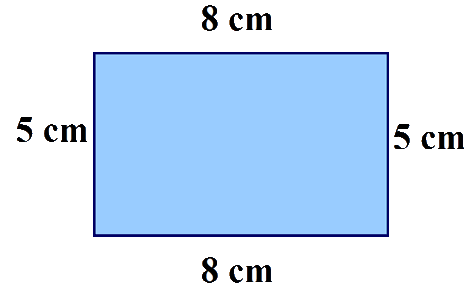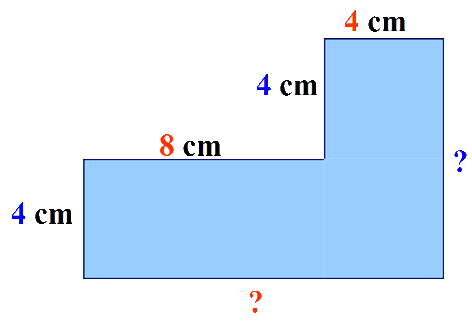# Shape and Space - Perimeters of 2D Shapes

## The Perimeter of a shape is the total distance all the way around the outside edge of a 2D shape.

### To find the perimeter of a shape, we need to know the lengths of all the sides of the shape and then simply add them together.## 8 + 6 + 4 + 6 = 24 cm

### Sometimes you will not be told the measurements of all the sides and you may have to work them out. Take this shape for example.You are not given the dimensions of the base or the right hand side.

But by looking at the two upper sides, you can see that if you added these together, they would equal the length of the base, 8 + 4 = 12 cm.

And by looking at the two left hand sides, you can see that if you added these together, they would equal the length of the right hand side, 4 + 4 = 8 cm.

You now know the dimensions of all the sides, so simply add them together,

## 4 + 8 + 4 + 4 + 8 + 12 = 40 cm

For shapes which have identical length sides, such as squares, equilateral triangles and regular polygons, you only need to know the dimensions of one side and then you can
multiply this amount by the number of sides.

Try these worksheets from Primary Resources.